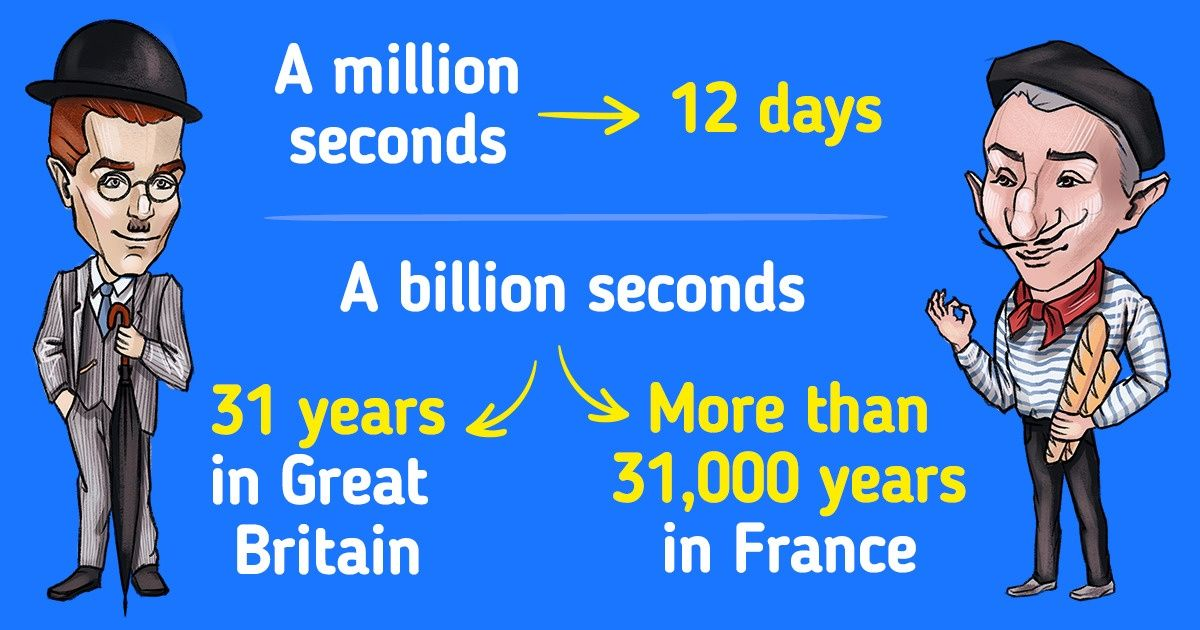# What to Call Big Numbers and Why It’s Different in Some Countries

Even children can easily tell 100 from 1000, and 1000 from 1 million. But not even all adults know that 1,000 million is 1 billion.

5-Minute Crafts is going to remind you what to call big numbers and why it’s different for different countries.

### What big numbers are called

For many people, it’s easy to imagine 1 billion. It might seem that the difference between 1 million and 1 billion is not that big. But look at these numbers from a different angle to change your point of view. For example:

• Million seconds — just 12 days
• Billion seconds — 31 years

It’s much harder to imagine and name numbers that are more than a billion. It’s also easy to make a mistake when you realize that the same numbers are called different things in different languages because most countries use 2 similar systems for naming big numbers.

### Short scale

One of the systems is called the short scale.

The names of the numbers are formed this way:

• At the beginning of the word, there’s a Latin number for the power that is added to the thousand.
• And then, “-illion” is added.

So, 1 million is 1000 in the power of 2, or 1,0001 + 1, one billion (milliard) — 1,0001 + 2, and 1 trillion is 1,0001 + 3. And so on.

To find out the number of zeros in a big number, you need to use the formula 3n + 3 where n is the number of the power of the number name added to the 1st power of the thousand (where n ≥ 1).

Example: Trillion is 1 0001 + 3. Use the formula and you get this: 3n + 3 = 3 × 3 + 3 = 9 + 3 = 12. So, a trillion is 1 with 12 zeros or 1,000,000,000,000, or 1012.

### Long scale

The other system is called the long scale. The names are formed this way:

• At the beginning of the word, there’s a Latin number for the power of a million.
• The next multiplier after -illion is -illiard — every “-illiard” word is one thousand times as large as the previous “-illion” word.

To find out the name of zeros in the -illion word, use the 6n formula. To find out the name of zeros in the -illiard word, use the 6n+3 formula.

Example: Billiard is a thousand billion. Use the formula and get 2 instead of n. You get: 6n + 3 = 6 × 2 + 3 = 12 + 3 = 15. So, a billiard is 1 with 15 zeros or 1,000,000,000,000,000, or 1015.

### What to pay attention to when working with a short or a long scale

There are numbers that don’t have their own names. For example, 10is 1 million. But 107 doesn’t have its own name, so we say “10 million.”

A lot depends on the system we use. For example, 6 sextillion in the short scale is 6,000 trillion or 6 trilliard in the long scale.

So, the same names can mean different numbers depending on the scale:

• 1,000,000,000, or 10is a billion (milliard) in some countries.
• 1,000,000,000,000, or 1012 is a billion in other countries.

If you see a number in a book, you have to consider when it was released if it’s from Great Britain. The thing is, before 1974, Great Britain used the long scale but then they switched to the short scale. This is why some films or movies released before 1974 might be translated incorrectly.

So, a million seconds is almost 12 days in both Britain and France but a billion seconds in Britain is 31 years, and in France, it’s over 31,000 years.

5-Minute Crafts/World/What to Call Big Numbers and Why It’s Different in Some Countries